Trieste tutorial: Metadynamics simulations with PLUMED

# Aims

The aim of this tutorial is to train users to perform metadynamics simulations with PLUMED, analyze the results, calculating free-energies as a function of the collective variables used, and estimating the associated error.

# Objectives

Once this tutorial is completed students will be able to:

• Write the PLUMED input file to perform metadynamics simulations
• Calculate the free energy from a metadynamics run
• Compute the error associated to the reconstructed free energy
• Evaluate the convergence of a metadynamics simulation
• Assess the choice of the collective variables

# Resources

The for this project contains the following files:

• diala.pdb: a PDB file for alanine dipeptide in vacuo
• topol.tpr: a GROMACS run file to perform MD of alanine dipeptide
• do_block_fes.py: a python script to perform error analysis

This tutorial has been tested on a pre-release version of version 2.4. However, it should not take advantage of 2.4-only features, thus should also work with version 2.3.

Note
We suggest to run the three exercises in three separate directories. For Exercise 3, you will need the output of the first two exercizes, so don't delete it!

# Introduction

We have seen that PLUMED can be used to compute collective variables. However, PLUMED is most often use to add forces on the collective variables. To this aim, we have implemented a variety of possible biases acting on collective variables. The complete documentation for all the biasing methods available in PLUMED can be found at the Bias page. In the following we will see how to build an adaptive bias potential with metadynamics. Here you can find a brief recap of the metadynamics theory.

We will play with a toy system, alanine dipeptide simulated in vacuo using the AMBER99SB-ILDN force field (see Fig. trieste-4-ala-fig). This rather simple molecule is useful to benchmark data analysis and free-energy methods. This system is a nice example because it presents two metastable states separated by a high free-energy barrier. It is conventional use to characterize the two states in terms of Ramachandran dihedral angles, which are denoted with $$\Phi$$ and $$\Psi$$ in Fig. trieste-4-transition-fig .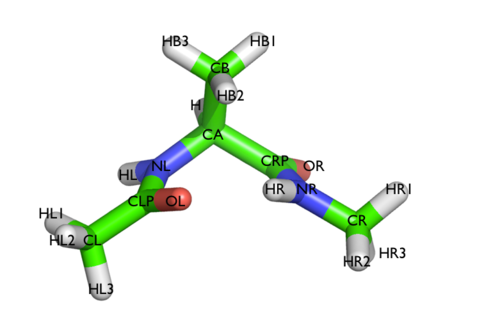The molecule of the day: alanine dipeptide.Two metastable states of alanine dipeptide are characterized by their Ramachandran dihedral angles.

# Exercise 1: my first metadynamics calculation

## Exercise 1a: setup and run

In this excercise we will setup and perform a well-tempered metadynamics run using the backbone dihedral $$\phi$$ as collective variable. During the calculation, we will also monitor the behavior of the other backbone dihedral $$\psi$$.

Here you can find a sample plumed.dat file that you can use as a template. Whenever you see an highlighted FILL string, this is a string that you should replace.

# Compute the backbone dihedral angle phi, defined by atoms C-N-CA-C
phi: TORSION ATOMS=__FILL__
# Compute the backbone dihedral angle psi, defined by atoms N-CA-C-N
psi: TORSION ATOMS=__FILL__

# Activate well-tempered metadynamics in phi
# Deposit a Gaussian every 500 time steps, with initial height equal to 1.2 kJoule/mol
PACE=500 HEIGHT=1.2
# the bias factor should be wisely chosen
BIASFACTOR=__FILL__
# Gaussian width (sigma) should be chosen based on CV fluctuation in unbiased run
SIGMA=__FILL__
# Gaussians will be written to file and also stored on grid
FILE=HILLS GRID_MIN=-pi GRID_MAX=pi
...

# Print both collective variables and the value of the bias potential on COLVAR file
PRINT ARG=__FILL__ FILE=COLVAR STRIDE=10


The syntax for the command METAD is simple. The directive is followed by a keyword ARG followed by the labels of the CVs on which the metadynamics potential will act. The keyword PACE determines the stride of Gaussian deposition in number of time steps, while the keyword HEIGHT specifies the height of the Gaussian in kJoule/mol. For each CVs, one has to specify the width of the Gaussian by using the keyword SIGMA. Gaussian will be written to the file indicated by the keyword FILE.

In this example, the bias potential will be stored on a grid, whose boundaries are specified by the keywords GRID_MIN and GRID_MAX. Notice that you can provide either the number of bins for every collective variable (GRID_BIN) or the desired grid spacing (GRID_SPACING). In case you provide both PLUMED will use the most conservative choice (highest number of bins) for each dimension. In case you do not provide any information about bin size (neither GRID_BIN nor GRID_SPACING) and if Gaussian width is fixed, PLUMED will use 1/5 of the Gaussian width as grid spacing. This default choice should be reasonable for most applications.

Once your plumed.dat file is complete, you can run a 10-ns long metadynamics simulations with the following command

> gmx mdrun -s topol.tpr -nsteps 5000000 -plumed plumed.dat


During the metadynamics simulation, PLUMED will create two files, named COLVAR and HILLS. The COLVAR file contains all the information specified by the PRINT command, in this case the value of the CVs every 10 steps of simulation, along with the current value of the metadynamics bias potential. We can use gnuplot to visualize the behavior of the CV during the simulation, as reported in the COLVAR file:

gnuplot> p "COLVAR" u 1:2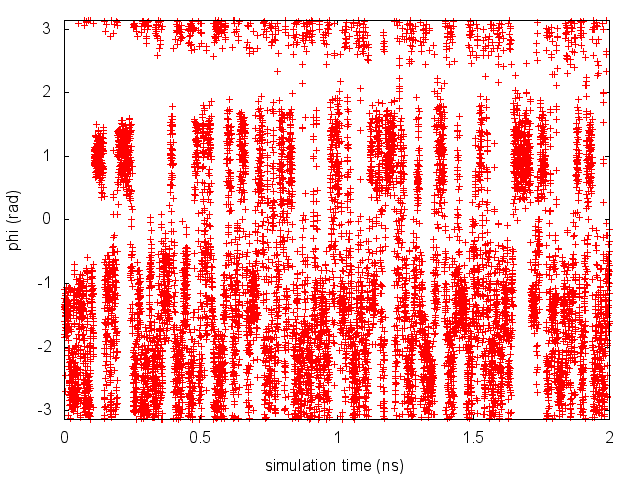Time evolution of the metadynamics CV during the first 2 ns of a metadynamics simulation of alanine dipeptide in vacuum.

By inspecting Figure trieste-4-phi-fig, we can see that the system is initialized in one of the two metastable states of alanine dipeptide. After a while (t=0.1 ns), the system is pushed by the metadynamics bias potential to visit the other local minimum. As the simulation continues, the bias potential fills the underlying free-energy landscape, and the system is able to diffuse in the entire phase space.

The HILLS file contains a list of the Gaussians deposited along the simulation. If we give a look at the header of this file, we can find relevant information about its content:

#! FIELDS time phi sigma_phi height biasf
#! SET multivariate false
#! SET min_phi -pi
#! SET max_phi pi


The line starting with FIELDS tells us what is displayed in the various columns of the HILLS file: the simulation time, the instantaneous value of $$\phi$$, the Gaussian width and height, and the biasfactor. We can use the HILLS file to visualize the decrease of the Gaussian height during the simulation, according to the well-tempered recipe: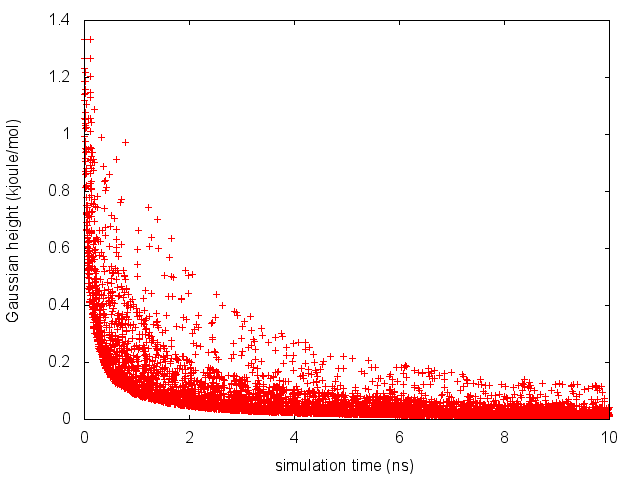Time evolution of the Gaussian height.

If we look carefully at the scale of the y-axis, we will notice that in the beginning the value of the Gaussian height is higher than the initial height specified in the input file, which should be 1.2 kJoule/mol. In fact, this column reports the height of the Gaussian rescaled by the pre-factor that in well-tempered metadynamics relates the bias potential to the free energy.

## Exercise 1b: estimating the free energy

One can estimate the free energy as a function of the metadynamics CVs directly from the metadynamics bias potential. In order to do so, the utility sum_hills should be used to sum the Gaussians deposited during the simulation and stored in the HILLS file.
To calculate the free energy as a function of $$\phi$$, it is sufficient to use the following command line:

plumed sum_hills --hills HILLS


The command above generates a file called fes.dat in which the free-energy surface as function of $$\phi$$ is calculated on a regular grid. One can modify the default name for the free energy file, as well as the boundaries and bin size of the grid, by using the following options of sum_hills :

--outfile - specify the outputfile for sumhills
--min - the lower bounds for the grid
--max - the upper bounds for the grid
--bin - the number of bins for the grid
--spacing - grid spacing, alternative to the number of bins


The result should look like this: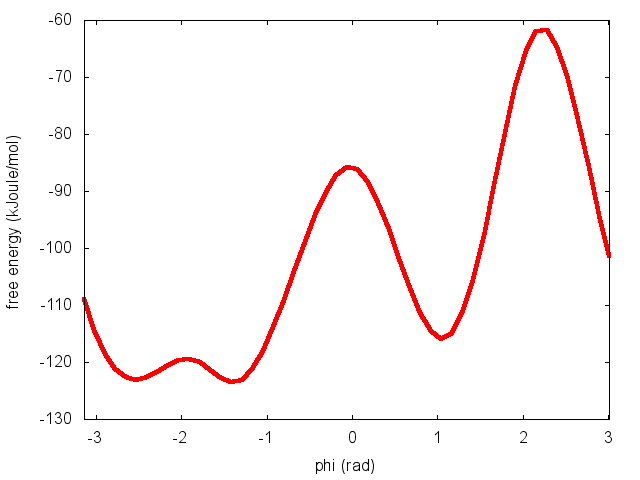Estimate of the free energy as a function of the dihedral phi from a 10ns-long well-tempered metadynamics simulation.

To assess the convergence of a metadynamics simulation, one can calculate the estimate of the free energy as a function of simulation time. At convergence, the reconstructed profiles should be similar. The option --stride should be used to give an estimate of the free energy every N Gaussians deposited, and the option --mintozero can be used to align the profiles by setting the global minimum to zero. If we use the following command line:

plumed sum_hills --hills HILLS --stride 100 --mintozero


one free energy is calculated every 100 Gaussians deposited, and the global minimum is set to zero in all profiles. The resulting plot should look like the following: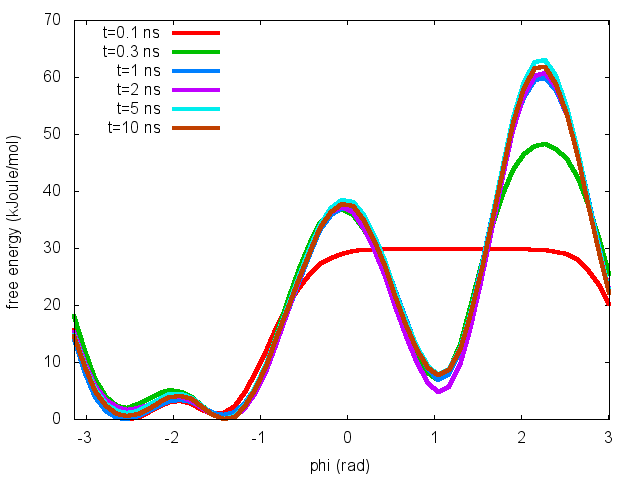Estimates of the free energy as a function of the dihedral phi calculated every 100 Gaussians deposited.

These two qualitative observations:

suggest that the simulation most likely converged.

Warning
The fact that the Gaussian height is decreasing to zero should not be used as a measure of convergence of your metadynamics simulation!
Note
The two observations above are necessary, but qualitative conditions for convergence. A quantitative assessment of convergence can be obtained by performing an error analysis of the reconstructed free-energy profile, as explained in the last exercise

# Exercise 2: playing with collective variables

In this exercise, we will run a well-tempered metadynamics simulation on alanine dipeptide in vacuum, this time using as CV the backbone dihedral $$\psi$$. Please complete the template plumed.dat file used in the previous exercise to run this calculation.

Once your plumed.dat file is complete, you can run a 10-ns long metadynamics simulations with the following command

> gmx mdrun -s topol.tpr -nsteps 5000000 -plumed plumed.dat


As we did in the previous exercise, we can use COLVAR to visualize the behavior of the CV during the simulation. Here we will plot at the same time the evolution of the metadynamics CV $$\psi$$ and of the other dihedral $$\phi$$.

gnuplot> p "COLVAR" u 1:2, "" u 1:3Time evolution of the dihedrals phi and psi during a 10-ns long metadynamics simulation using psi as CV.

By inspecting Figure trieste-4-metad-psi-phi-fig, we notice that something different happened compared to the previous exercise. At first the behavior of $$\psi$$ looks diffusive in the entire CV space. However, around t=1 ns, $$\psi$$ seems trapped in a region of the CV space in which it was previously diffusing without problems. The reason is that the non-biased CV $$\phi$$ after a while has jumped into a different local minima. Since $$\phi$$ is not directly biased, one has to wait for this (slow) degree of freedom to equilibrate before the free energy along $$\psi$$ can converge.

Try to repeat the analysis done in the previous exercise, i.e. calculate the estimate of the free energy as a function of time, first step to assess the convergence of this metadynamics simulation.

# Exercise 3: estimating the error in free-energies using block-analysis

In this exercise, we will calculate the error associated to the free-energy reconstructed by a well-tempered metadynamics simulation. The free energy and the errors will be calculated using the block-analysis technique explained in a previous lesson (Trieste tutorial: Averaging, histograms and block analysis). The procedure can be used to estimate the error in the free-energy as a function of the collective variable(s) used in the metadynamics simulation, or for any other function of the coordinates of the system.

First, we will calculate the "unbiasing" weights associated to each conformation sampled during the metadynamics run. In order to calculate these weights, we can use either of these two approaches:

1) Weights are calculated by considering the time-dependence of the metadynamics bias potential ;

2) Weights are calculated using the metadynamics bias potential obtained at the end of the simulation and assuming a constant bias during the entire course of the simulation .

In this exercise we will use the umbrella-sampling-like reweighting approach (Method 2).

To calculate the weights, we need to use the PLUMED driver utility and read the HILLS file along with the GROMACS trajectory file produced during the metadynamics simulation. Let's consider the metadynamics simulation carried out in Exercise 1. We need to prepare the plumed.dat input file to use in combination with driver. Here you can find a sample plumed.dat file that you can use as a template. Whenever you see an highlighted FILL string, this is a string that you should replace.

# Read old Gaussians deposited on HILLS file
RESTART
# Compute the backbone dihedral angle phi, defined by atoms C-N-CA-C
phi: TORSION ATOMS=__FILL__
# Compute the backbone dihedral angle psi, defined by atoms N-CA-C-N
psi: TORSION ATOMS=__FILL__

# Activate well-tempered metadynamics in phi
# Set the deposition stride to a large number
PACE=10000000 HEIGHT=1.2 BIASFACTOR=__FILL__
# Gaussian width (sigma) should be chosen based on CV fluctuation in unbiased run
SIGMA=__FILL__
# Gaussians will be read from file and stored on grid
FILE=HILLS GRID_MIN=-pi GRID_MAX=pi
...

# Print both collective variables and the value of the bias potential on COLVAR file
PRINT ARG=__FILL__ FILE=COLVAR STRIDE=1


Once your plumed.dat file is complete, you can use the driver utility to back-calculated the quantites needed for the error calculation

plumed driver --plumed plumed.dat --mf_xtc traj_comp.xtc


The COLVAR file produced by driver should look like this:

#! FIELDS time phi psi metad.bias
#! SET min_phi -pi
#! SET max_phi pi
#! SET min_psi -pi
#! SET max_psi pi
0.000000 0.907347 -0.144312 103.117323
1.000000 0.814296 -0.445819 100.974351
2.000000 1.118951 -0.909782 104.329630
3.000000 1.040781 -0.991788 104.559590
4.000000 1.218571 -1.020024 102.744053


Please check your plumed.dat file if your output looks different! Once the final bias has been evaluated on the entire metadynamics simulations, we can easily calculate the "unbiasing weights" using the umbrella-sampling-like approach:

# find maximum value of bias
bmax=awk 'BEGIN{max=0.}{if($1!="#!" &&$4>max)max=$4}END{print max}' COLVAR # print phi values and weights awk '{if($1!="#!") print $2,exp(($4-bmax)/kbt)}' kbt=2.494339 bmax=$bmax COLVAR > phi.weight  If you inspect the phi.weight file, you will see that each line contains the value of the dihedral $$\phi$$ along with the corresponding weight: 0.907347 0.0400579 0.814296 0.0169656 1.118951 0.0651276 1.040781 0.0714174 1.218571 0.0344903 1.090823 0.0700568 1.130800 0.0622998  At this point we can apply the block-analysis technique we have learned in the Trieste tutorial: Averaging, histograms and block analysis tutorial to calculate for different block sizes the average free-energy and the error. For your convenience, you can use the do_block_fes.py python script to read the phi.weight file and produce the desired output. We use a bash loop to use block sizes ranging from 1 to 1000: for i in seq 1 10 1000; do python3 do_block_fes.py phi.weight 1 -3.141593 3.018393 51 2.494339$i; done


For each value of block length N, you will obtain a separate fes.N.dat file, containing the value of the $$\phi$$ variable on a grid, the average free-energy, and the associated error (in Kjoule/mol):

   -3.141593       23.184653     0.080659
-3.018393       17.264462     0.055181
-2.895194       13.360259     0.047751
-2.771994       10.772696     0.043548
-2.648794        9.403544     0.042022


Finally, we can calculate the average error along the free-energy profile as a function of the block length:

for i in seq 1 10 1000; do a=awk '{tot+=$3}END{print tot/NR}' fes.$i.dat; echo $i$a; done > err.blocks


and visualize it using gnuplot:

gnuplot> p "err.blocks" u 1:2 w lp


As expected, the error increases with the block length until it reaches a plateau in correspondence of a dimension of the block that exceeds the correlation between data points (Fig. trieste-4-block-phi).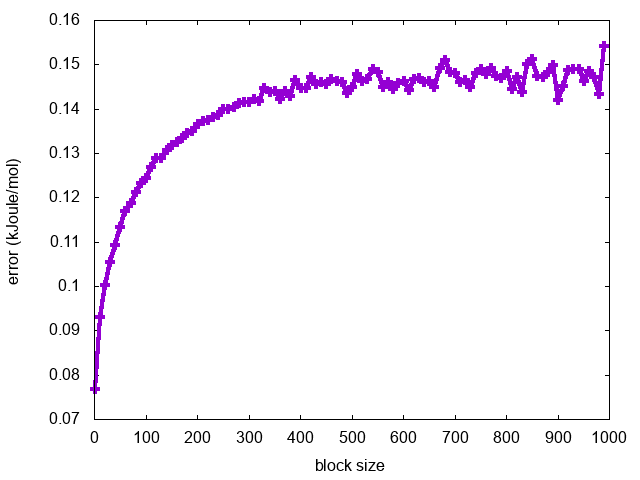Block analysis of a metadynamics simulation using phi as CV

To complete this exercise, you should do the following:

• calculate the error associated to the free energy as a function of the collective variable $$\psi$$ from Exercise 1
• calculate the error associated to the free energy as a function of the collective variable $$\psi$$ from Exercise 2
• compare the different behaviors in Exercise 1 and 2

What can we learn from this analysis about the convergence of the two metadynamics simulations and the quality of the collective variables chosen?

At this time, the most important question of this lecture becomes:

• Could we distinguish the different behavior (in terms of convergence) of the simulations in Exercise 1 and 2 simply by looking at the time series of the Gaussian height?

# Conclusions

In summary, in this tutorial you should have learned how to use PLUMED to:

• Setup and run a metadynamics calculation.
• Compute free energies from the metadynamics bias potential using the sum_hills utility.
• Calculate the error in the reconstructed free energy using block analysis.
• Discriminate between good and bad collective variables.
• Evaluate the convergence of a metadynamics simulation.